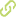# Modeling the Phenanthrene Decomposition Adsorbed in Soil by Ozone: Model Characterization and Experimental Validation•
• Overview
•
• Identity
•
•
• View All
•

### Abstract

• © 2015 Springer International Publishing. This paper analyzes the mathematical modeling procedure to describe the decomposition of adsorbed phenanthrene in prototypical and real soil samples (sand and agricultural soil, respectively) by ozone. The modeling scheme considered a set of ordinary differential equations with time varying coefficients. This model used the adsorbed ozone in the soil, the ozone reacting with the contaminant and the phenanthrene concentration in the soil sample. The main parameters involved in the mathematical model included a time varying ozone saturation function (k sat (t)) and reaction constants (k r). These parameters were calculated using the ozone concentration variation at the reactor output, named as ozonogram, and the measurements of phenanthrene decomposition through ozonation. The model was validated using two series of experiments: (1) soil saturated with ozone in the absence of the contaminant and (2) soil artificially contaminated with phenanthrene. In both cases, the proposed parametric identification method yields to validate the mathematical model. This fact was confirmed by the correspondence between numerical simulations and experimental data. In particular, total decomposition of phenanthrene adsorbed in two different systems (ozone-sand and ozone-agricultural soil) was obtained after 15 and 30 min of reaction, respectively. This difference was obtained as a consequence of soil physicochemical characteristics: specific surface area and pore volume. The ozonation reaction rate constants of phenanthrene in the sand and agricultural soil were calculated using the same parameter identification scheme.

### Publication date

• June 29, 2015